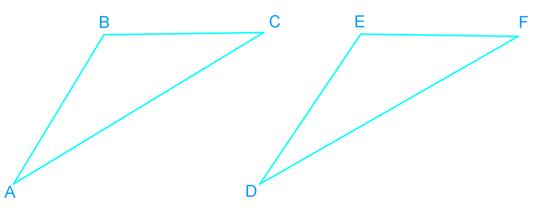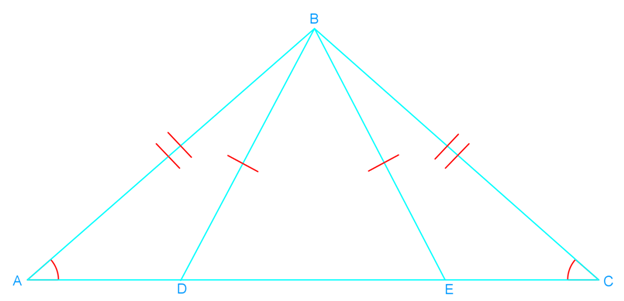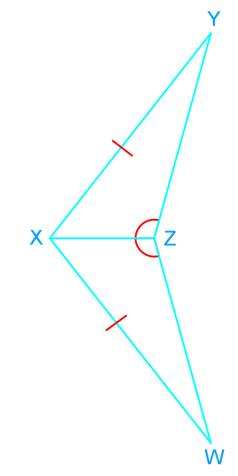# Triangles congruent by ASA and AAS proofs#### Everything You Need in One Place

Homework problems? Exam preparation? Trying to grasp a concept or just brushing up the basics? Our extensive help & practice library have got you covered.#### Learn and Practice With Ease

Our proven video lessons ease you through problems quickly, and you get tonnes of friendly practice on questions that trip students up on tests and finals.#### Instant and Unlimited Help

Our personalized learning platform enables you to instantly find the exact walkthrough to your specific type of question. Activate unlimited help now!

0/1
##### Intros
###### Lessons
1. Similar tirangles VS. Congruent triangles
2. Ways to prove congruency:
• SSS
• SAS
• ASA
• AAS
0/5
##### Examples
###### Lessons
1. What is the third congruence that is needed to prove that $\triangle ABC$$\cong$$\triangle DEF$ using the given postulate or theorem?1. $\overline {AB}$$\cong$$\overline {DE}$ and $\angle C$$\cong$$\angle F$
Prove by AAS.
2. $\overline {AC}$$\cong$$\overline {DF}$ and $\angle A$$\cong$$\angle D$
Prove by ASA.
3. $\angle A$$\cong$$\angle D$ and $\angle B$$\cong$$\angle E$
Prove by AAS.
2. Decide if the following triangles are congruent. Show your reasoning.
1. $\triangle ABC$, $\triangle CBD$2. $\triangle XYZ$, $\triangle XWZ$